# Multi-Class Fault Detection Using Simulated Data

This example shows how to use a Simulink model to generate fault and healthy data. The data is used to develop a multi-class classifier to detect different combinations of faults. The example uses a triplex reciprocating pump model and includes leak, blocking, and bearing faults.

### Setup the Model

This example uses many supporting files that are stored in a zip file. Unzip the file to get access to the supporting files, load the model parameters, and create the reciprocating pump library.

```if ~exist('+mech_hydro_forcesPS','dir') unzip('pdmRecipPump_supportingfiles.zip') end % Load Parameters pdmRecipPump_Parameters %Pump CAT_Pump_1051_DataFile_imported %CAD % Create Simscape library if needed if exist('mech_hydro_forcesPS_Lib','file')~=4 ssc_build mech_hydro_forcesPS end```

### Reciprocating Pump Model

The reciprocating pump consists of an electric motor, the pump housing, pump crank and pump plungers.

```mdl = 'pdmRecipPump'; open_system(mdl)```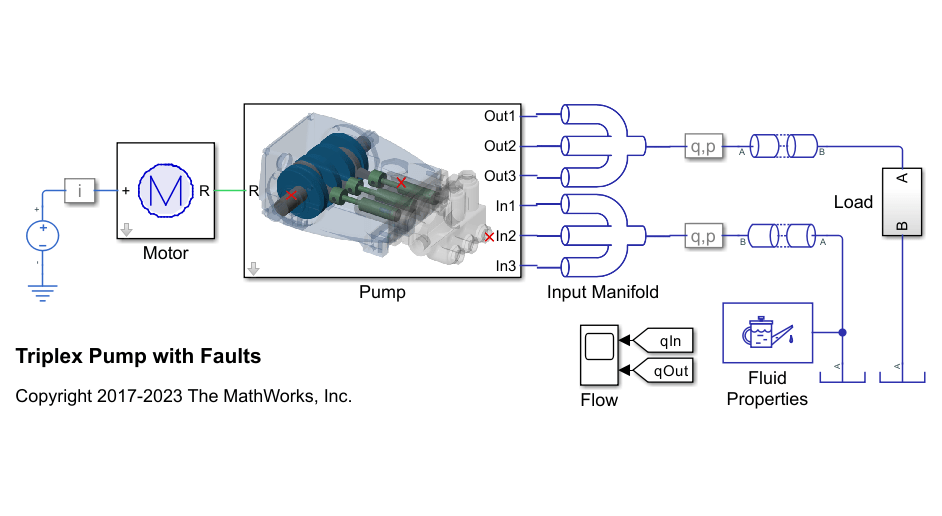`open_system([mdl,'/Pump'])`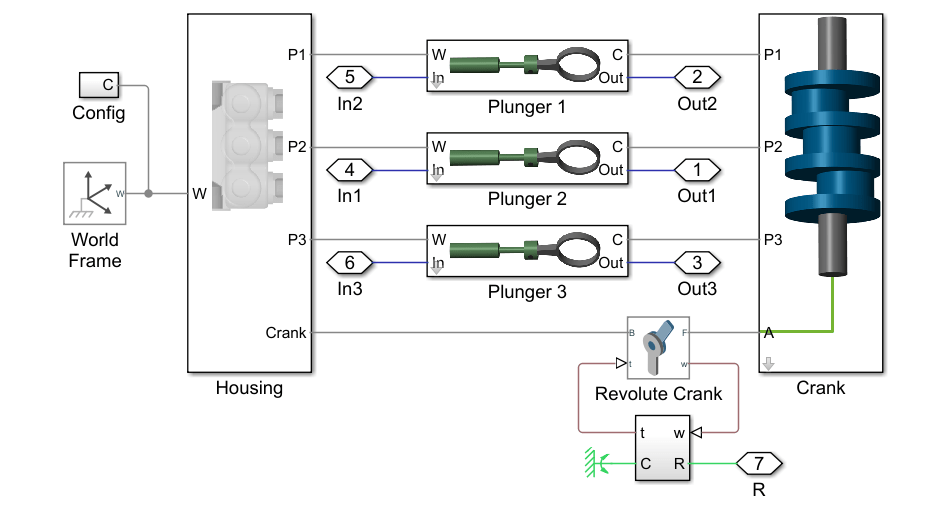The pump model is configured to model three types of faults; cylinder leaks, blocked inlet, and increased bearing friction. These faults are parameterized as workspace variables and configured through the pump block dialog.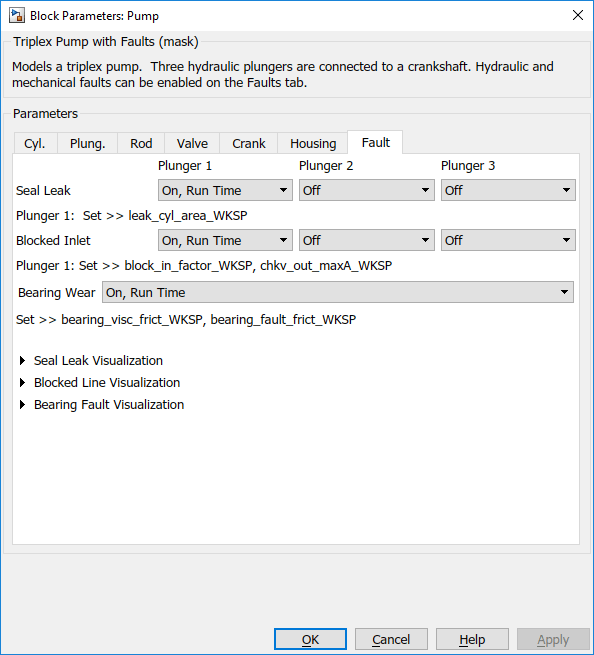### Simulating Fault and Healthy Data

For each of the three fault types create an array of values that represent the fault severity, ranging from no fault to a significant fault.

```% Define fault parameter variations numParValues = 10; leak_area_set_factor = linspace(0.00,0.036,numParValues); leak_area_set = leak_area_set_factor*TRP_Par.Check_Valve.In.Max_Area; leak_area_set = max(leak_area_set,1e-9); % Leakage area cannot be 0 blockinfactor_set = linspace(0.8,0.53,numParValues); bearingfactor_set = linspace(0,6e-4,numParValues);```

The pump model is configured to include noise, thus running the model with the same fault parameter values will result in different simulation outputs. This is useful for developing a classifier as it means there can be multiple simulation results for the same fault condition and severity. To configure simulations for such results, create vectors of fault parameter values where the values represent no faults, a single fault, combinations of two faults, and combinations of three faults. For each group (no fault, single fault, etc.) create 125 combinations of fault values from the fault parameter values defined above. This gives a total of 1000 combinations of fault parameter values. Note that running these 1000 simulations in parallel takes around an hour on a standard desktop and generates around 620MB of data. To reduce simulation time, reduce the number of fault combinations to 20 by changing `runAll = true` to `runAll = false. Note that a` larger dataset results in a more robust classifier.

```% Set number of elements in each fault group runAll = false; if runAll % Create a large dataset to build a robust classifier nPerGroup = 125; else % Create a smaller dataset to reduce simulation time nPerGroup = 20; %#ok<UNRCH> end % No fault simulations leakArea = repmat(leak_area_set(1),nPerGroup,1); blockingFactor = repmat(blockinfactor_set(1),nPerGroup,1); bearingFactor = repmat(bearingfactor_set(1),nPerGroup,1); % Single fault simulations idx = ceil(10*rand(nPerGroup,1)); leakArea = [leakArea; leak_area_set(idx)']; blockingFactor = [blockingFactor;repmat(blockinfactor_set(1),nPerGroup,1)]; bearingFactor = [bearingFactor;repmat(bearingfactor_set(1),nPerGroup,1)]; idx = ceil(10*rand(nPerGroup,1)); leakArea = [leakArea; repmat(leak_area_set(1),nPerGroup,1)]; blockingFactor = [blockingFactor;blockinfactor_set(idx)']; bearingFactor = [bearingFactor;repmat(bearingfactor_set(1),nPerGroup,1)]; idx = ceil(10*rand(nPerGroup,1)); leakArea = [leakArea; repmat(leak_area_set(1),nPerGroup,1)]; blockingFactor = [blockingFactor;repmat(blockinfactor_set(1),nPerGroup,1)]; bearingFactor = [bearingFactor;bearingfactor_set(idx)']; % Double fault simulations idxA = ceil(10*rand(nPerGroup,1)); idxB = ceil(10*rand(nPerGroup,1)); leakArea = [leakArea; leak_area_set(idxA)']; blockingFactor = [blockingFactor;blockinfactor_set(idxB)']; bearingFactor = [bearingFactor;repmat(bearingfactor_set(1),nPerGroup,1)]; idxA = ceil(10*rand(nPerGroup,1)); idxB = ceil(10*rand(nPerGroup,1)); leakArea = [leakArea; leak_area_set(idxA)']; blockingFactor = [blockingFactor;repmat(blockinfactor_set(1),nPerGroup,1)]; bearingFactor = [bearingFactor;bearingfactor_set(idxB)']; idxA = ceil(10*rand(nPerGroup,1)); idxB = ceil(10*rand(nPerGroup,1)); leakArea = [leakArea; repmat(leak_area_set(1),nPerGroup,1)]; blockingFactor = [blockingFactor;blockinfactor_set(idxA)']; bearingFactor = [bearingFactor;bearingfactor_set(idxB)']; % Triple fault simulations idxA = ceil(10*rand(nPerGroup,1)); idxB = ceil(10*rand(nPerGroup,1)); idxC = ceil(10*rand(nPerGroup,1)); leakArea = [leakArea; leak_area_set(idxA)']; blockingFactor = [blockingFactor;blockinfactor_set(idxB)']; bearingFactor = [bearingFactor;bearingfactor_set(idxC)'];```

Use the fault parameter combinations to create `Simulink.SimulationInput` objects. For each simulation input ensure that the random seed is set differently to generate different results.

```for ct = numel(leakArea):-1:1 simInput(ct) = Simulink.SimulationInput(mdl); simInput(ct) = setVariable(simInput(ct),'leak_cyl_area_WKSP',leakArea(ct)); simInput(ct) = setVariable(simInput(ct),'block_in_factor_WKSP',blockingFactor(ct)); simInput(ct) = setVariable(simInput(ct),'bearing_fault_frict_WKSP',bearingFactor(ct)); simInput(ct) = setVariable(simInput(ct),'noise_seed_offset_WKSP',ct-1); end```

Use the `generateSimulationEnsemble` function to run the simulations defined by the `Simulink.SimulationInput` objects defined above and store the results in a local sub-folder. Then create a `simulationEnsembleDatastore` from the stored results.

```% Run the simulation and create an ensemble to manage the simulation % results if isfolder('./Data') % Delete existing mat files delete('./Data/*.mat') end [ok,e] = generateSimulationEnsemble(simInput,fullfile('.','Data'),'UseParallel',true);```
```[08-Sep-2020 21:01:46] Checking for availability of parallel pool... Starting parallel pool (parpool) using the 'local' profile ... Connected to the parallel pool (number of workers: 6). [08-Sep-2020 21:02:27] Starting Simulink on parallel workers... [08-Sep-2020 21:02:55] Configuring simulation cache folder on parallel workers... [08-Sep-2020 21:02:55] Loading model on parallel workers... [08-Sep-2020 21:03:02] Transferring base workspace variables used in the model to parallel workers... [08-Sep-2020 21:03:08] Running simulations... [08-Sep-2020 21:04:21] Completed 1 of 160 simulation runs [08-Sep-2020 21:04:21] Completed 2 of 160 simulation runs [08-Sep-2020 21:04:22] Completed 3 of 160 simulation runs [08-Sep-2020 21:04:23] Completed 4 of 160 simulation runs [08-Sep-2020 21:04:24] Completed 5 of 160 simulation runs [08-Sep-2020 21:04:24] Completed 6 of 160 simulation runs [08-Sep-2020 21:04:47] Completed 7 of 160 simulation runs [08-Sep-2020 21:04:48] Completed 8 of 160 simulation runs [08-Sep-2020 21:04:49] Completed 9 of 160 simulation runs [08-Sep-2020 21:04:50] Completed 10 of 160 simulation runs [08-Sep-2020 21:04:52] Completed 11 of 160 simulation runs [08-Sep-2020 21:04:53] Completed 12 of 160 simulation runs [08-Sep-2020 21:05:14] Completed 13 of 160 simulation runs [08-Sep-2020 21:05:15] Completed 14 of 160 simulation runs [08-Sep-2020 21:05:17] Completed 15 of 160 simulation runs [08-Sep-2020 21:05:18] Completed 16 of 160 simulation runs [08-Sep-2020 21:05:20] Completed 17 of 160 simulation runs [08-Sep-2020 21:05:21] Completed 18 of 160 simulation runs [08-Sep-2020 21:05:41] Completed 19 of 160 simulation runs [08-Sep-2020 21:05:43] Completed 20 of 160 simulation runs [08-Sep-2020 21:05:45] Completed 21 of 160 simulation runs [08-Sep-2020 21:05:47] Completed 22 of 160 simulation runs [08-Sep-2020 21:05:49] Completed 23 of 160 simulation runs [08-Sep-2020 21:05:51] Completed 24 of 160 simulation runs [08-Sep-2020 21:06:08] Completed 25 of 160 simulation runs [08-Sep-2020 21:06:10] Completed 26 of 160 simulation runs [08-Sep-2020 21:06:13] Completed 27 of 160 simulation runs [08-Sep-2020 21:06:16] Completed 28 of 160 simulation runs [08-Sep-2020 21:06:18] Completed 29 of 160 simulation runs [08-Sep-2020 21:06:21] Completed 30 of 160 simulation runs [08-Sep-2020 21:06:34] Completed 31 of 160 simulation runs [08-Sep-2020 21:06:38] Completed 32 of 160 simulation runs [08-Sep-2020 21:06:41] Completed 33 of 160 simulation runs [08-Sep-2020 21:06:44] Completed 34 of 160 simulation runs [08-Sep-2020 21:06:48] Completed 35 of 160 simulation runs [08-Sep-2020 21:06:51] Completed 36 of 160 simulation runs [08-Sep-2020 21:07:01] Completed 37 of 160 simulation runs [08-Sep-2020 21:07:05] Completed 38 of 160 simulation runs [08-Sep-2020 21:07:09] Completed 39 of 160 simulation runs [08-Sep-2020 21:07:13] Completed 40 of 160 simulation runs [08-Sep-2020 21:07:17] Completed 41 of 160 simulation runs [08-Sep-2020 21:07:21] Completed 42 of 160 simulation runs [08-Sep-2020 21:07:32] Completed 43 of 160 simulation runs [08-Sep-2020 21:07:37] Completed 44 of 160 simulation runs [08-Sep-2020 21:07:41] Completed 45 of 160 simulation runs [08-Sep-2020 21:07:46] Completed 46 of 160 simulation runs [08-Sep-2020 21:07:50] Completed 47 of 160 simulation runs [08-Sep-2020 21:07:58] Completed 48 of 160 simulation runs [08-Sep-2020 21:08:04] Completed 49 of 160 simulation runs [08-Sep-2020 21:08:10] Completed 50 of 160 simulation runs [08-Sep-2020 21:08:16] Completed 51 of 160 simulation runs [08-Sep-2020 21:08:23] Completed 52 of 160 simulation runs [08-Sep-2020 21:08:33] Completed 53 of 160 simulation runs [08-Sep-2020 21:08:41] Completed 54 of 160 simulation runs [08-Sep-2020 21:08:51] Completed 55 of 160 simulation runs [08-Sep-2020 21:09:01] Completed 56 of 160 simulation runs [08-Sep-2020 21:09:11] Completed 57 of 160 simulation runs [08-Sep-2020 21:09:22] Completed 58 of 160 simulation runs [08-Sep-2020 21:09:34] Completed 59 of 160 simulation runs [08-Sep-2020 21:09:45] Completed 60 of 160 simulation runs [08-Sep-2020 21:09:57] Completed 61 of 160 simulation runs [08-Sep-2020 21:10:08] Completed 62 of 160 simulation runs [08-Sep-2020 21:10:16] Completed 63 of 160 simulation runs [08-Sep-2020 21:10:25] Completed 64 of 160 simulation runs [08-Sep-2020 21:10:36] Completed 65 of 160 simulation runs [08-Sep-2020 21:10:44] Completed 66 of 160 simulation runs [08-Sep-2020 21:10:57] Completed 67 of 160 simulation runs [08-Sep-2020 21:11:10] Completed 68 of 160 simulation runs [08-Sep-2020 21:11:21] Completed 69 of 160 simulation runs [08-Sep-2020 21:11:31] Completed 70 of 160 simulation runs [08-Sep-2020 21:11:44] Completed 71 of 160 simulation runs [08-Sep-2020 21:11:55] Completed 72 of 160 simulation runs [08-Sep-2020 21:12:11] Completed 73 of 160 simulation runs [08-Sep-2020 21:12:28] Completed 74 of 160 simulation runs [08-Sep-2020 21:12:43] Completed 75 of 160 simulation runs [08-Sep-2020 21:12:59] Completed 76 of 160 simulation runs [08-Sep-2020 21:13:12] Completed 77 of 160 simulation runs [08-Sep-2020 21:13:27] Completed 78 of 160 simulation runs [08-Sep-2020 21:13:43] Completed 79 of 160 simulation runs [08-Sep-2020 21:13:58] Completed 80 of 160 simulation runs [08-Sep-2020 21:14:18] Completed 81 of 160 simulation runs [08-Sep-2020 21:14:30] Completed 82 of 160 simulation runs [08-Sep-2020 21:14:36] Completed 83 of 160 simulation runs [08-Sep-2020 21:14:42] Completed 84 of 160 simulation runs [08-Sep-2020 21:14:47] Completed 85 of 160 simulation runs [08-Sep-2020 21:14:53] Completed 86 of 160 simulation runs [08-Sep-2020 21:14:59] Completed 87 of 160 simulation runs [08-Sep-2020 21:15:05] Completed 88 of 160 simulation runs [08-Sep-2020 21:15:12] Completed 89 of 160 simulation runs [08-Sep-2020 21:15:18] Completed 90 of 160 simulation runs [08-Sep-2020 21:15:26] Completed 91 of 160 simulation runs [08-Sep-2020 21:15:33] Completed 92 of 160 simulation runs [08-Sep-2020 21:15:40] Completed 93 of 160 simulation runs [08-Sep-2020 21:15:46] Completed 94 of 160 simulation runs [08-Sep-2020 21:15:53] Completed 95 of 160 simulation runs [08-Sep-2020 21:16:00] Completed 96 of 160 simulation runs [08-Sep-2020 21:16:07] Completed 97 of 160 simulation runs [08-Sep-2020 21:16:14] Completed 98 of 160 simulation runs [08-Sep-2020 21:16:21] Completed 99 of 160 simulation runs [08-Sep-2020 21:16:29] Completed 100 of 160 simulation runs [08-Sep-2020 21:16:36] Completed 101 of 160 simulation runs [08-Sep-2020 21:16:41] Completed 102 of 160 simulation runs [08-Sep-2020 21:16:48] Completed 103 of 160 simulation runs [08-Sep-2020 21:16:53] Completed 104 of 160 simulation runs [08-Sep-2020 21:16:58] Completed 105 of 160 simulation runs [08-Sep-2020 21:17:02] Completed 106 of 160 simulation runs [08-Sep-2020 21:17:10] Completed 107 of 160 simulation runs [08-Sep-2020 21:17:15] Completed 108 of 160 simulation runs [08-Sep-2020 21:17:21] Completed 109 of 160 simulation runs [08-Sep-2020 21:17:26] Completed 110 of 160 simulation runs [08-Sep-2020 21:17:32] Completed 111 of 160 simulation runs [08-Sep-2020 21:17:37] Completed 112 of 160 simulation runs [08-Sep-2020 21:17:43] Completed 113 of 160 simulation runs [08-Sep-2020 21:17:48] Completed 114 of 160 simulation runs [08-Sep-2020 21:17:54] Completed 115 of 160 simulation runs [08-Sep-2020 21:18:00] Completed 116 of 160 simulation runs [08-Sep-2020 21:18:05] Completed 117 of 160 simulation runs [08-Sep-2020 21:18:12] Completed 118 of 160 simulation runs [08-Sep-2020 21:18:18] Completed 119 of 160 simulation runs [08-Sep-2020 21:18:24] Completed 120 of 160 simulation runs [08-Sep-2020 21:18:30] Completed 121 of 160 simulation runs [08-Sep-2020 21:18:36] Completed 122 of 160 simulation runs [08-Sep-2020 21:18:42] Completed 123 of 160 simulation runs [08-Sep-2020 21:18:49] Completed 124 of 160 simulation runs [08-Sep-2020 21:18:57] Completed 125 of 160 simulation runs [08-Sep-2020 21:19:03] Completed 126 of 160 simulation runs [08-Sep-2020 21:19:09] Completed 127 of 160 simulation runs [08-Sep-2020 21:19:14] Completed 128 of 160 simulation runs [08-Sep-2020 21:19:20] Completed 129 of 160 simulation runs [08-Sep-2020 21:19:28] Completed 130 of 160 simulation runs [08-Sep-2020 21:19:35] Completed 131 of 160 simulation runs [08-Sep-2020 21:19:41] Completed 132 of 160 simulation runs [08-Sep-2020 21:19:47] Completed 133 of 160 simulation runs [08-Sep-2020 21:19:54] Completed 134 of 160 simulation runs [08-Sep-2020 21:20:03] Completed 135 of 160 simulation runs [08-Sep-2020 21:20:12] Completed 136 of 160 simulation runs [08-Sep-2020 21:20:19] Completed 137 of 160 simulation runs [08-Sep-2020 21:20:27] Completed 138 of 160 simulation runs [08-Sep-2020 21:20:35] Completed 139 of 160 simulation runs [08-Sep-2020 21:20:43] Completed 140 of 160 simulation runs [08-Sep-2020 21:20:50] Completed 141 of 160 simulation runs [08-Sep-2020 21:20:56] Completed 142 of 160 simulation runs [08-Sep-2020 21:21:03] Completed 143 of 160 simulation runs [08-Sep-2020 21:21:13] Completed 144 of 160 simulation runs [08-Sep-2020 21:21:19] Completed 145 of 160 simulation runs [08-Sep-2020 21:21:25] Completed 146 of 160 simulation runs [08-Sep-2020 21:21:32] Completed 147 of 160 simulation runs [08-Sep-2020 21:21:42] Completed 148 of 160 simulation runs [08-Sep-2020 21:21:49] Completed 149 of 160 simulation runs [08-Sep-2020 21:21:57] Completed 150 of 160 simulation runs [08-Sep-2020 21:22:05] Completed 151 of 160 simulation runs [08-Sep-2020 21:22:14] Completed 152 of 160 simulation runs [08-Sep-2020 21:22:22] Completed 153 of 160 simulation runs [08-Sep-2020 21:22:31] Completed 154 of 160 simulation runs [08-Sep-2020 21:22:39] Completed 155 of 160 simulation runs [08-Sep-2020 21:22:47] Completed 156 of 160 simulation runs [08-Sep-2020 21:22:55] Completed 157 of 160 simulation runs [08-Sep-2020 21:23:03] Completed 158 of 160 simulation runs [08-Sep-2020 21:23:12] Completed 159 of 160 simulation runs [08-Sep-2020 21:23:21] Completed 160 of 160 simulation runs [08-Sep-2020 21:23:23] Cleaning up parallel workers... ```
`ens = simulationEnsembleDatastore(fullfile('.','Data'));`

### Processing and Extracting Features from the Simulation Results

The model is configured to log the pump output pressure, output flow, motor speed and motor current.

`ens.DataVariables`
```ans = 8×1 string "SimulationInput" "SimulationMetadata" "iMotor_meas" "pIn_meas" "pOut_meas" "qIn_meas" "qOut_meas" "wMotor_meas" ```

For each member in the ensemble preprocess the pump output flow and compute condition indicators based on the pump output flow. The condition indicators are later used for fault classification. For preprocessing remove the first 0.8 seconds of the output flow as this contains transients from simulation and pump startup. As part of the preprocessing compute the power spectrum of the output flow, and use the SimulationInput to return the values of the fault variables.

Configure the ensemble so that the read only returns the variables of interest and call the `preprocess` function that is defined at the end of this example.

```ens.SelectedVariables = ["qOut_meas", "SimulationInput"]; reset(ens) data = read(ens)```
```data=1×2 table qOut_meas SimulationInput __________________ ______________________________ {2001×1 timetable} {1×1 Simulink.SimulationInput} ```
`[flow,flowP,flowF,faultValues] = preprocess(data);`

Plot the flow and flow spectrum. The plotted data is for a fault free condition.

```% Figure with nominal subplot(211); plot(flow.Time,flow.Data); subplot(212); semilogx(flowF,pow2db(flowP)); xlabel('Hz')```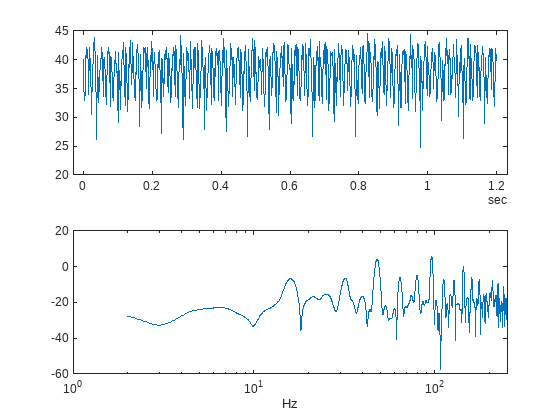The flow spectrum reveals resonant peaks at expected frequencies. Specifically, the pump motor speed is 950 rpm, or 15.833 Hz, and since the pump has 3 cylinders the flow is expected to have a fundamental at 3*15.833 Hz, or 47.5 Hz, as well as harmonics at multiples of 47.5 Hz. The flow spectrum clearly shows the expected resonant peaks. Faults in one cylinder of the pump will result in resonances at the pump motor speed, 15.833 Hz and its harmonics.

The flow spectrum and slow signal gives some ideas of possible condition indicators. Specifically, common signal statistics such as mean, variance, etc. as well as spectrum characteristics. Spectrum condition indicators relating to the expected harmonics such as the frequency with the peak magnitude, energy around 15.833 Hz, energy around 47.5 Hz, energy above 100 Hz, are computed. The frequency of the spectral kurtosis peak is also computed.

Configure the ensemble with data variables for the condition indicators and condition variables for fault variable values. Then call the `extractCI` function to compute the features, and use the `writeToLastMemberRead` command to add the feature and fault variable values to the ensemble. The `extractCI` function is defined at the end of this example.

```ens.DataVariables = [ens.DataVariables; ... "fPeak"; "pLow"; "pMid"; "pHigh"; "pKurtosis"; ... "qMean"; "qVar"; "qSkewness"; "qKurtosis"; ... "qPeak2Peak"; "qCrest"; "qRMS"; "qMAD"; "qCSRange"]; ens.ConditionVariables = ["LeakFault","BlockingFault","BearingFault"]; feat = extractCI(flow,flowP,flowF); dataToWrite = [faultValues, feat]; writeToLastMemberRead(ens,dataToWrite{:})```

The above code preprocesses and computes the condition indicators for the first member of the simulation ensemble. Repeat this for all the members in the ensemble using the ensemble `hasdata` command. To get an idea of the simulation results under different fault conditions plot every hundredth element of the ensemble.

```%Figure with nominal and faults figure, subplot(211); lFlow = plot(flow.Time,flow.Data,'LineWidth',2); subplot(212); lFlowP = semilogx(flowF,pow2db(flowP),'LineWidth',2); xlabel('Hz') ct = 1; lColors = get(lFlow.Parent,'ColorOrder'); lIdx = 2; % Loop over all members in the ensemble, preprocess % and compute the features for each member while hasdata(ens) % Read member data data = read(ens); % Preprocess and extract features from the member data [flow,flowP,flowF,faultValues] = preprocess(data); feat = extractCI(flow,flowP,flowF); % Add the extracted feature values to the member data dataToWrite = [faultValues, feat]; writeToLastMemberRead(ens,dataToWrite{:}) % Plot member signal and spectrum for every 100th member if mod(ct,100) == 0 line('Parent',lFlow.Parent,'XData',flow.Time,'YData',flow.Data, ... 'Color', lColors(lIdx,:)); line('Parent',lFlowP.Parent,'XData',flowF,'YData',pow2db(flowP), ... 'Color', lColors(lIdx,:)); if lIdx == size(lColors,1) lIdx = 1; else lIdx = lIdx+1; end end ct = ct + 1; end```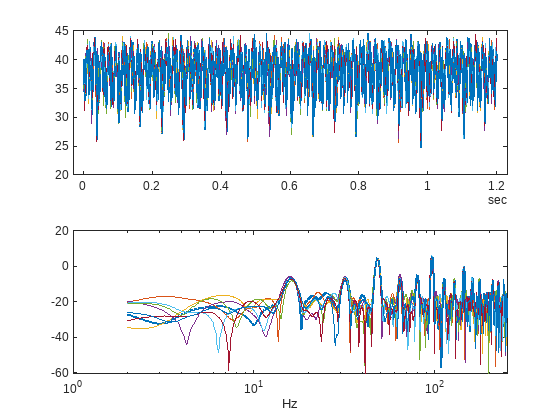Note that under different fault conditions and severities the spectrum contains harmonics at the expected frequencies.

### Detect and Classify Pump Faults

The previous section preprocessed and computed condition indicators from the flow signal for all the members of the simulation ensemble, which correspond to the simulation results for different fault combinations and severities. The condition indicators can be used to detect and classify pump faults from a pump flow signal.

Configure the simulation ensemble to read the condition indicators, and use the tall and gather commands to load all the condition indicators and fault variable values into memory

```% Get data to design a classifier. reset(ens) ens.SelectedVariables = [... "fPeak","pLow","pMid","pHigh","pKurtosis",... "qMean","qVar","qSkewness","qKurtosis",... "qPeak2Peak","qCrest","qRMS","qMAD","qCSRange",... "LeakFault","BlockingFault","BearingFault"]; idxLastFeature = 14; % Load the condition indicator data into memory data = gather(tall(ens));```
```Evaluating tall expression using the Parallel Pool 'local': - Pass 1 of 1: 0% complete Evaluation 0% complete ```
```- Pass 1 of 1: Completed in 11 sec Evaluation completed in 11 sec ```
`data(1:10,:)`
```ans=10×17 table fPeak pLow pMid pHigh pKurtosis qMean qVar qSkewness qKurtosis qPeak2Peak qCrest qRMS qMAD qCSRange LeakFault BlockingFault BearingFault ______ _______ ______ ______ _________ ______ ______ _________ _________ __________ ______ ______ ______ ________ _________ _____________ ____________ 43.909 0.84932 117.83 18.867 276.49 35.573 7.5235 -0.73064 2.778 13.86 1.1491 35.679 2.2319 42692 1e-09 0.8 0 43.909 0.46288 126.04 18.969 12.417 35.577 7.8766 -0.70939 2.63 13.336 1.1451 35.688 2.3235 42699 1e-09 0.8 0 44.03 66.853 151.07 23.624 230.13 34.357 9.4974 -0.54522 2.8046 15.243 1.1665 34.495 2.4703 41231 1.6e-06 0.71 0 40.699 6.0325 89.768 21.02 252.48 32.617 6.7424 -0.70295 2.7538 12.264 1.1457 32.721 2.1208 39139 4e-07 0.8 6.6667e-05 8.6012 14.366 29.295 9.8881 485.93 18.623 9.325 -0.02553 2.0546 14.315 1.3422 18.871 2.6236 22347 2.8e-06 0.8 0.0006 43.969 33.122 141.94 24.284 154.8 34.682 8.6405 -0.53746 2.7521 15.692 1.1955 34.807 2.3614 41620 1.2e-06 0.8 0 9.5096 25.219 31.388 13.27 65.397 21.352 6.7393 -0.25342 2.4885 13.704 1.2738 21.509 2.1357 25619 2e-06 0.8 0.00046667 26.83 1.114 31.794 15.963 434.6 21.432 3.6724 -0.44013 2.6938 10.304 1.2108 21.517 1.5589 25716 4e-07 0.8 0.00053333 9.0252 16.051 23.779 12.955 302.15 20.006 7.5907 -0.13753 2.2392 14.006 1.2837 20.195 2.3023 24010 2.4e-06 0.8 0.00053333 43.969 12.291 124.8 22.447 478.48 34.986 8.0954 -0.71245 2.9268 15.015 1.1585 35.101 2.2984 41987 8e-07 0.8 0 ```

The fault variable values for each ensemble member (row in the data table) can be converted to fault flags and the fault flags combined to single flag that captures the different fault status of each member.

```% Convert the fault variable values to flags data.LeakFlag = data.LeakFault > 1e-6; data.BlockingFlag = data.BlockingFault < 0.8; data.BearingFlag = data.BearingFault > 0; data.CombinedFlag = data.LeakFlag+2*data.BlockingFlag+4*data.BearingFlag;```

Create a classifier that takes as input the condition indicators and returns the combined fault flag. Train a support vector machine that uses a 2nd order polynomial kernel. Use the `cvpartition` command to partition the ensemble members into a set for training and a set for validation.

```rng('default') % for reproducibility predictors = data(:,1:idxLastFeature); response = data.CombinedFlag; cvp = cvpartition(size(predictors,1),'KFold',5); % Create and train the classifier template = templateSVM(... 'KernelFunction', 'polynomial', ... 'PolynomialOrder', 2, ... 'KernelScale', 'auto', ... 'BoxConstraint', 1, ... 'Standardize', true); combinedClassifier = fitcecoc(... predictors(cvp.training(1),:), ... response(cvp.training(1),:), ... 'Learners', template, ... 'Coding', 'onevsone', ... 'ClassNames', [0; 1; 2; 3; 4; 5; 6; 7]);```

Check the performance of the trained classifier using the validation data and plot the results on a confusion plot.

```% Check performance by computing and plotting the confusion matrix actualValue = response(cvp.test(1),:); predictedValue = predict(combinedClassifier, predictors(cvp.test(1),:)); confdata = confusionmat(actualValue,predictedValue); figure, labels = {'None', 'Leak','Blocking', 'Leak & Blocking', 'Bearing', ... 'Bearing & Leak', 'Bearing & Blocking', 'All'}; h = heatmap(confdata, ... 'YLabel', 'Actual leak fault', ... 'YDisplayLabels', labels, ... 'XLabel', 'Predicted fault', ... 'XDisplayLabels', labels, ... 'ColorbarVisible','off');```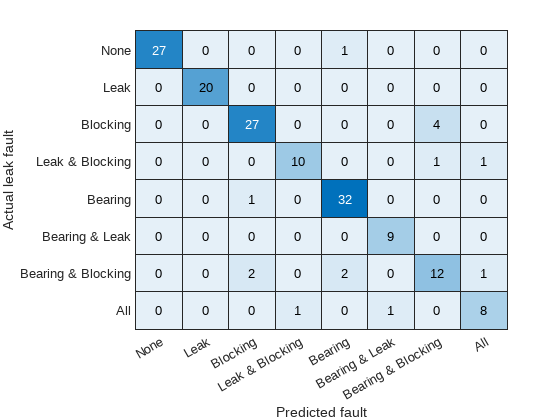The confusion plot shows for each combination of faults the number of times the fault combination was correctly predicted (the diagonal entries of the plot) and the number of times the fault combination was incorrectly predicted (the off-diagonal entries).

The confusion plot shows that the classifier did not correctly classify some fault conditions (the off diagonal terms). However, the no fault condition was correctly predicted. In a couple of places a no fault condition was predicted when there was a fault (the first column), otherwise a fault was predicted although it may not be exactly the correct fault condition. Overall the validation accuracy was 84% and the accuracy at predicting that there is a fault 98%.

```% Compute the overall accuracy of the classifier sum(diag(confdata))/sum(confdata(:))```
```ans = 0.5000 ```
```% Compute the accuracy of the classifier at predicting % that there is a fault 1-sum(confdata(2:end,1))/sum(confdata(:))```
```ans = 0.9375 ```

Examine the cases where no fault was predicted but a fault did exist. First find cases in the validation data where the actual fault was a blocking fault but a no fault was predicted.

```vData = data(cvp.test(1),:); b1 = (actualValue==2) & (predictedValue==0); fData = vData(b1,15:17)```
```fData=2×3 table LeakFault BlockingFault BearingFault _________ _____________ ____________ 1e-09 0.74 0 8e-07 0.59 0 ```

Find cases in the validation data where the actual fault was a bearing fault but a no fault was predicted.

```b2 = (actualValue==4) & (predictedValue==0); vData(b2,15:17)```
```ans = 0×3 empty table ```

Examining the cases where no fault was predictive but a fault did exist reveals that they occur when the blocking fault value of 0.77 is close to its nominal value of 0.8, or the bearing fault value of 6.6e-5 is close to its nominal value of 0. Plotting the spectrum for the case with a small blocking fault value and comparing with a fault free condition reveals that spectra are very similar making detection difficult. Re-training the classifier but including a blocking value of 0.77 as a non fault condition would significantly improve the performance of the fault detector. Alternatively, using additional pump measurements could provide more information and improve the ability to detect small blocking faults.

```% Configure the ensemble to only read the flow and fault variable values ens.SelectedVariables = ["qOut_meas","LeakFault","BlockingFault","BearingFault"]; reset(ens) % Load the ensemble member data into memory data = gather(tall(ens));```
```Evaluating tall expression using the Parallel Pool 'local': - Pass 1 of 1: 0% complete Evaluation 0% complete ```
```- Pass 1 of 1: Completed in 9.7 sec Evaluation completed in 9.8 sec ```
```% Look for the member that was incorrectly predicted, and % compute its power spectrum idx = ... data.BlockingFault == fData.BlockingFault(1) & ... data.LeakFault == fData.LeakFault(1) & ... data.BearingFault == fData.BearingFault(1); flow1 = data(idx,1); flow1 = flow1.qOut_meas{1}; [flow1P,flow1F] = pspectrum(flow1); % Look for a member that does not have any faults idx = ... data.BlockingFault == 0.8 & ... data.LeakFault == 1e-9 & ... data.BearingFault == 0; flow2 = data(idx,1); flow2 = flow2.qOut_meas{1}; [flow2P,flow2F] = pspectrum(flow2); % Plot the power spectra semilogx(... flow1F,pow2db(flow1P),... flow2F,pow2db(flow2P)); xlabel('Hz') legend('Small blocking fault','No fault')```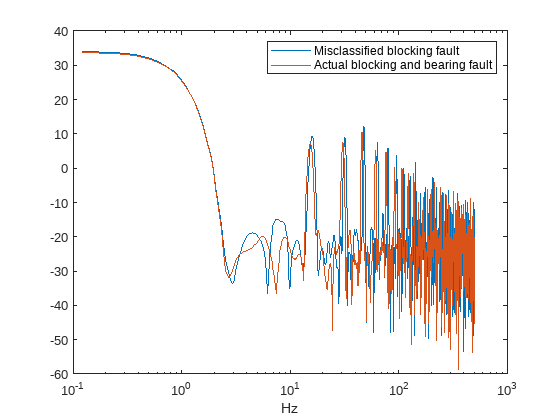### Conclusion

This example showed how to use a Simulink model to model faults in a reciprocating pump, simulate the model under different fault combinations and severities, extract condition indicators from the pump output flow and use the condition indicators to train a classifier to detect pump faults. The example examined the performance of fault detection using the classifier and noted that small blocking faults are very similar to the no fault condition and cannot be reliably detected.

### Supporting Functions

```function [flow,flowSpectrum,flowFrequencies,faultValues] = preprocess(data) % Helper function to preprocess the logged reciprocating pump data. % Remove the 1st 0.8 seconds of the flow signal tMin = seconds(0.8); flow = data.qOut_meas{1}; flow = flow(flow.Time >= tMin,:); flow.Time = flow.Time - flow.Time(1); % Ensure the flow is sampled at a uniform sample rate flow = retime(flow,'regular','linear','TimeStep',seconds(1e-3)); % Remove the mean from the flow and compute the flow spectrum fA = flow; fA.Data = fA.Data - mean(fA.Data); [flowSpectrum,flowFrequencies] = pspectrum(fA,'FrequencyLimits',[2 250]); % Find the values of the fault variables from the SimulationInput simin = data.SimulationInput{1}; vars = {simin.Variables.Name}; idx = strcmp(vars,'leak_cyl_area_WKSP'); LeakFault = simin.Variables(idx).Value; idx = strcmp(vars,'block_in_factor_WKSP'); BlockingFault = simin.Variables(idx).Value; idx = strcmp(vars,'bearing_fault_frict_WKSP'); BearingFault = simin.Variables(idx).Value; % Collect the fault values in a cell array faultValues = {... 'LeakFault', LeakFault, ... 'BlockingFault', BlockingFault, ... 'BearingFault', BearingFault}; end function ci = extractCI(flow,flowP,flowF) % Helper function to extract condition indicators from the flow signal % and spectrum. % Find the frequency of the peak magnitude in the power spectrum. pMax = max(flowP); fPeak = flowF(flowP==pMax); % Compute the power in the low frequency range 10-20 Hz. fRange = flowF >= 10 & flowF <= 20; pLow = sum(flowP(fRange)); % Compute the power in the mid frequency range 40-60 Hz. fRange = flowF >= 40 & flowF <= 60; pMid = sum(flowP(fRange)); % Compute the power in the high frequency range >100 Hz. fRange = flowF >= 100; pHigh = sum(flowP(fRange)); % Find the frequency of the spectral kurtosis peak [pKur,fKur] = pkurtosis(flow); pKur = fKur(pKur == max(pKur)); % Compute the flow cumulative sum range. csFlow = cumsum(flow.Data); csFlowRange = max(csFlow)-min(csFlow); % Collect the feature and feature values in a cell array. % Add flow statistic (mean, variance, etc.) and common signal % characteristics (rms, peak2peak, etc.) to the cell array. ci = {... 'qMean', mean(flow.Data), ... 'qVar', var(flow.Data), ... 'qSkewness', skewness(flow.Data), ... 'qKurtosis', kurtosis(flow.Data), ... 'qPeak2Peak', peak2peak(flow.Data), ... 'qCrest', peak2rms(flow.Data), ... 'qRMS', rms(flow.Data), ... 'qMAD', mad(flow.Data), ... 'qCSRange',csFlowRange, ... 'fPeak', fPeak, ... 'pLow', pLow, ... 'pMid', pMid, ... 'pHigh', pHigh, ... 'pKurtosis', pKur(1)}; end ```